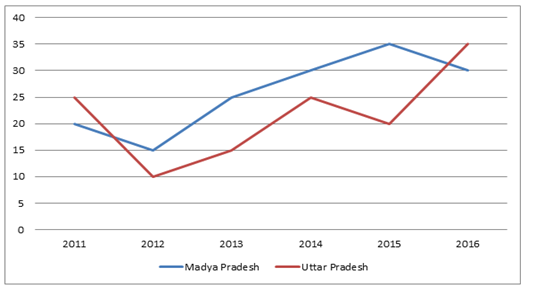# “20-20” Quantitative Aptitude | Crack SBI Clerk 2018 Day-5

Dear Friends, SBI Clerk 2018 Notification has been released we hope you all have started your preparation. Here we have started New Series of Practice Materials specially for SBI Clerk 2018. Aspirants those who are preparing for the exams can use this “20-20” Quantitative Aptitude Questions.

[WpProQuiz 1142]

Click “Start Quiz” to attend these Questions and view Explanation

### Click Here for SBI Clerk Prelims and Mains 2018 – Full Length Mock Test

Directions (Q. 1-5): Study the graph carefully to answer the questions that follow, the bar graph shows the percentage increase of population of two states.1. What is the difference between the percentage increase in percent rise of population of Uttar Pradesh in the year 2016 from the previous year and the percentage increase in percent rise of population of Madya Pradesh in the year 2013 from the previous year?
1. 8.66
2. 9.99
3. 15.66
4. 8.33
5. None of these
1. What is the average percent rise in population of Madya Pradesh over all the years together?
1. 21 1/3
2. 24 1/3
3. 26 5/6
4. 27 5/6
5. None of these
1. If the population in Uttar Pradesh in the year 2015 was 3. 63 lakhs, what was the population in same state in the year 2013?
1. 2.86 lakhs
2. 2.28 lakhs
3. 2.42 lakhs
4. 2.64 lakhs
5. 2.52 lakhs
1. If the population in Madya Pradesh in the year 2012 was 1.84 lakhs, what was the population in the same state in the year 2013?
1. 2.25 lakhs
2. 2.30 lakhs
3. 2.17 lakhs
4. 2.20 lakhs
5. None of these
1. If the population in Madya Pradesh in the year 2015 was 4.695 lakhs and the population in Uttar Pradesh in the year 2015 was 4.36 lakhs, then what was the ratio of population of both states in the year 2016?
1. 305 : 294
2. 294 : 305
3. 301 : 293
4. 302 : 297
5. 305 : 299

Directions (Q. 6–10): What should come in place of the question mark (?) in the following questions?

6). (1 / 13) × 3237 + (3 / 14) × 5362 + 200% of 1 = ? + 1335

1. 15
2. 35
3. 55
4. 65
5. 75

7). (11 / 7) of (5 / 8) of (13 / 9) of 8568 = ?

1. 12310
2. 12155
3. 12265
4. 12450
5. 12255

8). 200.1 × 9.9 – 25 × 62.5 + 12 × 144 = ? – 26.49

1. 1627.98
2. 1842.28
3. 1958.8
4. 1972.88
5. 2172.98

9).(608.40 × ?) ÷ 225 + 37 = 375

1. 115
2. 135
3. 130
4. 124
5. None of these

10).12.25 × 7.2 + 84.33 =?

1. 182.51
2. 177.44
3. 174.33
4. 172.53
5. None of these

Directions (Q. 11-15): What should come in place of question mark (?) in the following number series:

11). 17,   9,   15,   40,   143.5,   ?

1. 505.75
2. 578.5
3. 650.25
4. 578
5. 678.5

12). 3,   5,   13,   49,   241,   ?

1. 1210
2. 1451
3. 1221
4. 1441
5. 1200

13). 508,   256,   130,   67,   35.5,   ?

1. 18.25
2. 19.75
3. 17.25
4. 15.75
5. 17.75

14). 543,   668,   704,   1047,   ?,   1840

1. 1110
2. 1111
3. 1115
4. 1121
5. 1112

15). 71,   78,   149,   227,   376,   603, ?

1. 779
2. 879
3. 980
4. 979
5. 880
1. The average profit earned by a trader in the first three days of a week is Rs.6750. The average loss for the next two days is Rs.3850. What should he the average loss/ gain per day for the last two days so that there is no loss or gain in the whole week?
1. Rs.6275
2. Rs.6325
3. Rs.6250
4. Rs.6725
5. Other than the given options
1. 15 Men can finish a piece of work in 60 days. 12 men started working and in 45 days they finished a certain part of the work. If it is required to finish the remaining work in 8 days, how many men must be added to the existing workforce?
1. 48
2. 38
3. 33
4. 36
5. 44

18). In how many different ways can 5 girls and 4 boys be seated in a row so that they come alternately?

1. 2880
2. 3000
3. 2580
4. 2780
5. None of these

19). The ratio of A’s to B’s salary is 4 : 9. If B’s salary is increased by 45%, then his salary becomes Rs.33930. What is the salary of A?

1. Rs.5000
2. Rs.5500
3. Rs.5200
4. Rs.8200
5. None of these

20).The simple interest accrued on an amount of Rs. 18500 at the end of three years is Rs. 6150. What would be the compound interest accrued on the same amount at the same rate of interest for the same period?

1. Rs. 6801.17
2. Rs. 6900
3. Rs. 7000
4. Rs. 7801.23
5. None of these